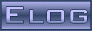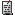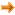Back Midas Rome Roody Rootana
 Midas DAQ System Not logged in17 Jun 2019, Konstantin Olchanski, Bug Fix, removed modbset() from mhttpd.js17 Jun 2019, Stefan Ritt, Bug Fix, removed modbset() from mhttpd.js17 Jun 2019, Konstantin Olchanski, Bug Fix, removed modbset() from mhttpd.js17 Jun 2019, Konstantin Olchanski, Bug Fix, restored modbset() in midas.js17 Jun 2019, Stefan Ritt, Bug Fix, removed modbset() from mhttpd.js18 Jun 2019, Konstantin Olchanski, Bug Fix, removed modbset() from mhttpd.js18 Jun 2019, Stefan Ritt, Bug Fix, removed modbset() from mhttpd.js18 Jun 2019, Konstantin Olchanski, Bug Fix, removed modbset() from mhttpd.js
Message ID: 1560     Entry time: 18 Jun 2019     In reply to: 1559     Reply to this: 1561
 Author: Konstantin Olchanski Topic: Bug Fix Subject: removed modbset() from mhttpd.js
```> A ladder of promise event handlers is certainly one possibility to enforce the order of ODB writes, but I wonder if we could so something simpler:
>
> - modbset creates an object remembering the status of the RPC request. Initially, this object receives the status "open request"
> - when the rpc call got executed successfully, the callback sets the state of the above object to "request succeeded" or "request failed" (in case of error)
> - if a new modbset comes BEFORE the previous one has completed, the function queues the new request in a data field of the above object
> - if a rpc call finishes, and a queued new rpc request is present, it gets executed
>
> This would be relatively easy to be implemented and keep the order of the rpc calls. Does that make sense?
>

Yes, this is a neat idea, I am really happy with how a complete rpc request can be held by one object, and we can make queues of them, etc.

Anyhow here is the proof of the pudding. I added a test to example.html, there are two buttons, one makes 5 modbset() calls, second has a ladder of 5 db_paste calls. Then I watch
the result in odbedit. 1, 2, 3, 4, 5 is the modbset(), 6, 7, 8, 9, 10 is the ladder of db_paste calls:

\$ odbedit
[local:javascript1:S]/>watch Example/int
Watch key "/Example/int" to be modified, abort with any key

/Example/int = 1
/Example/int = 2
/Example/int = 3
/Example/int = 4
/Example/int = 5

/Example/int = 1
/Example/int = 5 <== fault
/Example/int = 5
/Example/int = 5
/Example/int = 5

/Example/int = 1
/Example/int = 2
/Example/int = 3
/Example/int = 5 <== 4 and 5 reversed
/Example/int = 4 <== 4 and 5 reversed

/Example/int = 6
/Example/int = 7
/Example/int = 8
/Example/int = 9
/Example/int = 10

/Example/int = 6
/Example/int = 8 <== should be 7
/Example/int = 8
/Example/int = 9
/Example/int = 10

/Example/int = 6
/Example/int = 7
/Example/int = 8
/Example/int = 9
/Example/int = 10

I immediately notice that we have a race condition between the RPCs, db_watch notifications and db_get_value() in the watch handler:
there are 5 rpcs, 5 watch notifications, 5 calls to db_get_value() in the watch handler, but sometimes the handler is too slow
and the data in odb changes before it reads it, thus duplicate values (missing "7" above). (The old db_open_record() had a "hidden"
db_get_value() inside it, while db_watch() requires an explicit db_get_value() call, making it obvious why we get

Possible fixes for this is to slow down the RPCs (the race condition is still there, probability is reduced) or send the changed
data as part of the notification. If this were C/C++, a "sleep(1)" between modbset() calls would have fixed it,
but there is no sleeping and waiting in javascript. (I guess one could use a ladder of timers).

Other than that, I am surprised how easy it was to see that indeed out-of-order RPCs can happen, see the case
of out-of-order 4 and 5 above. It only took me maybe 5-10 clicks on the button to see that. I expected that I would
need to try several browsers or use a slow network connection, but here it is, on my home mac, localhost network,

Below is the test code. I do NOT vote that everybody should use ladders of db_paste calls.

function test_modbset() {
modbset("/example/int", 1);
modbset("/example/int", 2);
modbset("/example/int", 3);
modbset("/example/int", 4);
modbset("/example/int", 5);
}

function test_chained_db_paste() {
var paths = [ "/example/int" ];
mjsonrpc_db_paste(paths,).then(function(rpc) {
mjsonrpc_db_paste(paths,).then(function(rpc) {
mjsonrpc_db_paste(paths,).then(function(rpc) {
mjsonrpc_db_paste(paths,).then(function(rpc) {
mjsonrpc_db_paste(paths,).then(function(rpc) {
// nothing